•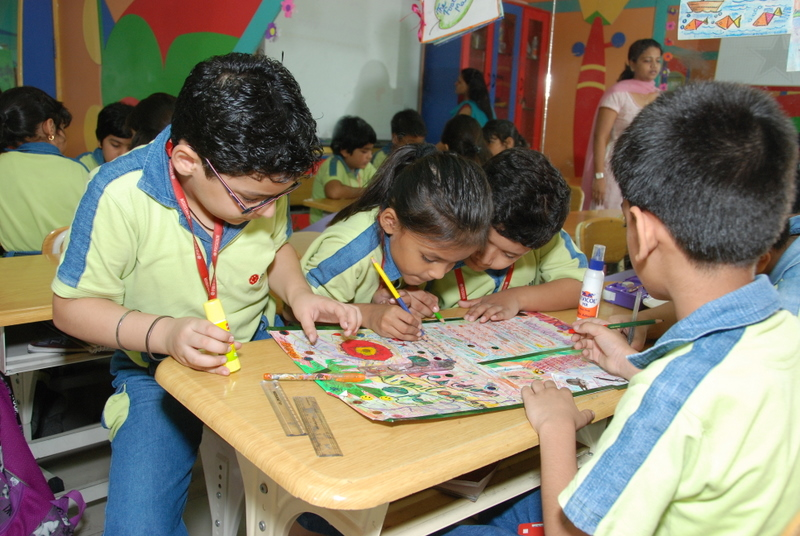TBL

•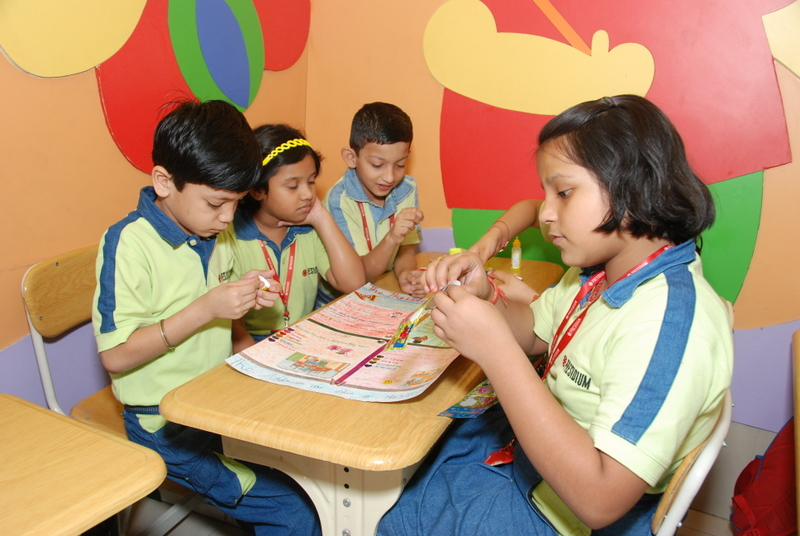TBL

•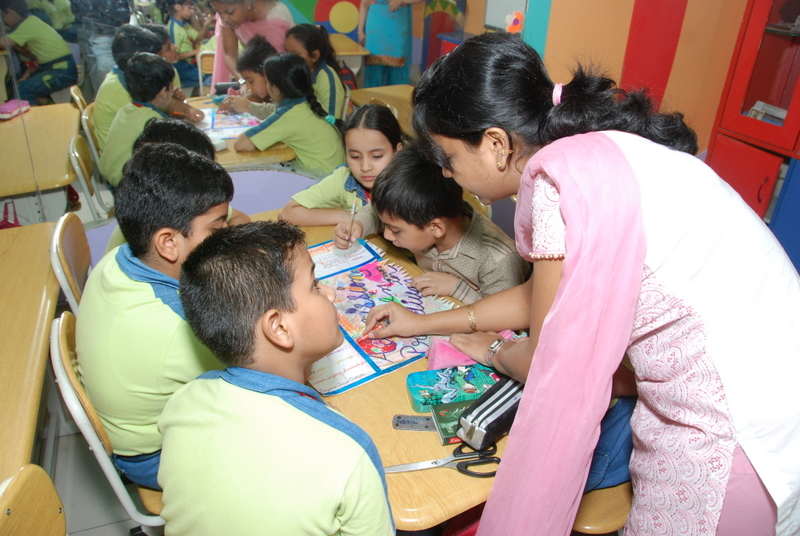TBL

•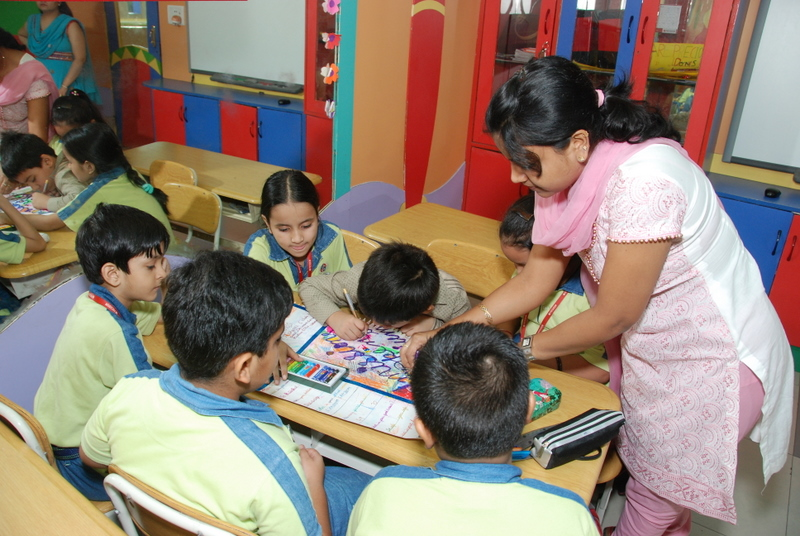TBL

•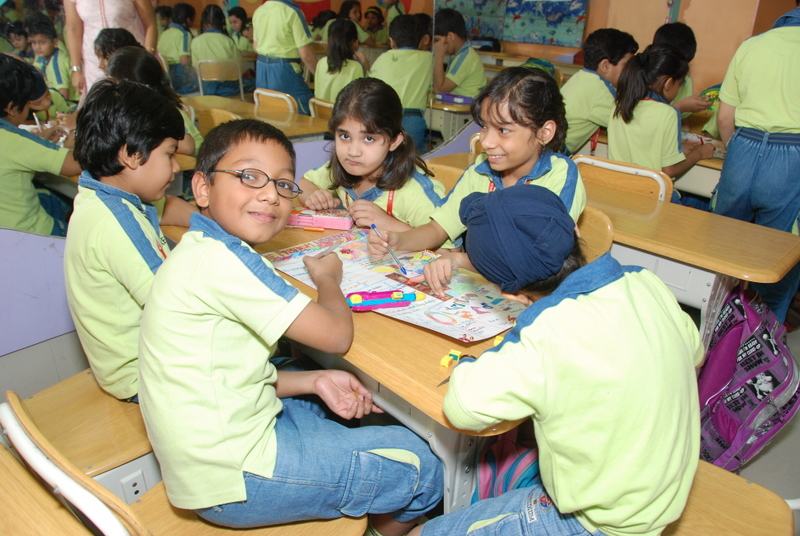TBL

•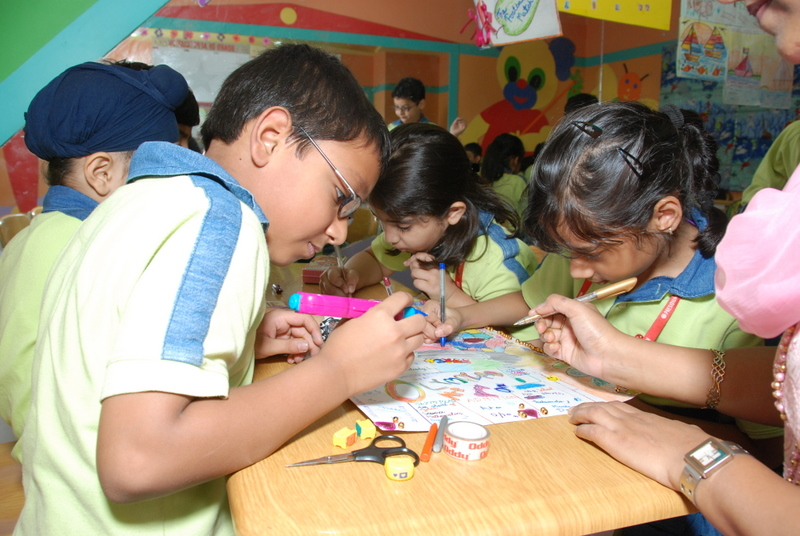TBL

•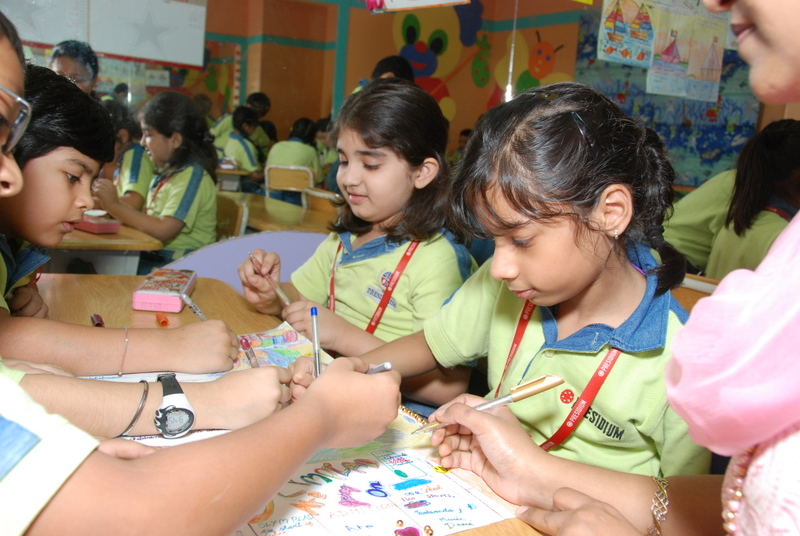TBL

•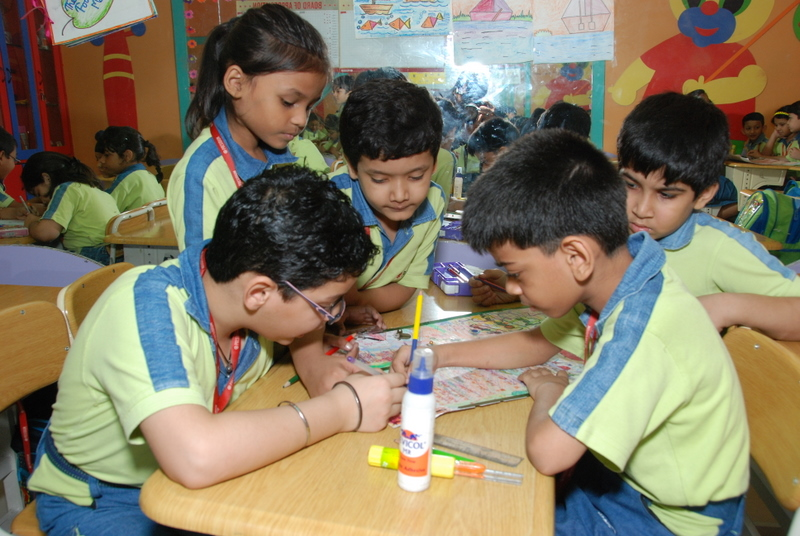TBL

•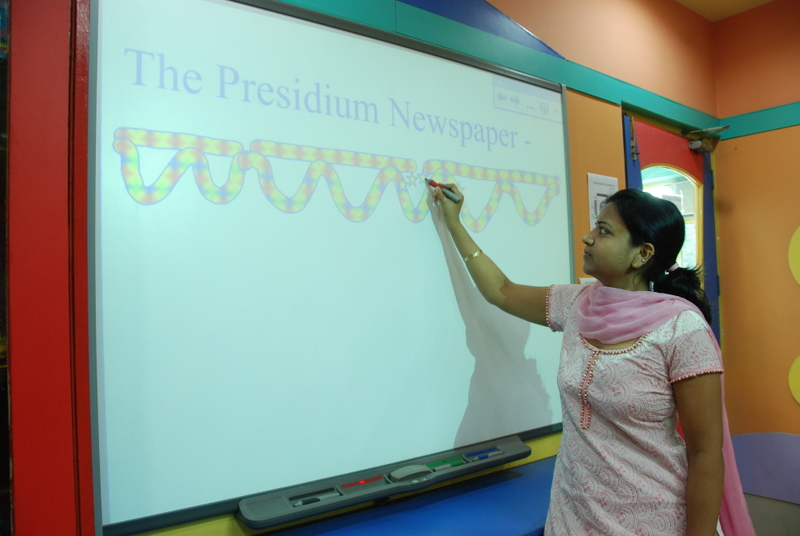TBL

•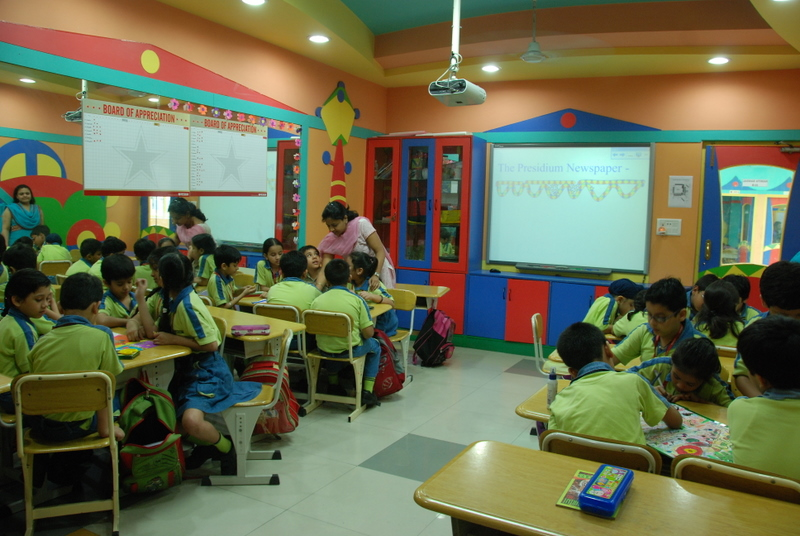TBL

•TBL

•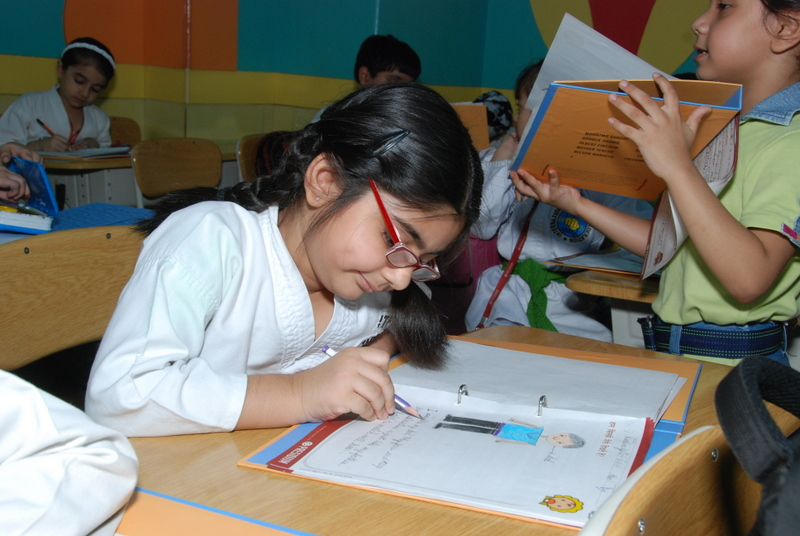TBL

•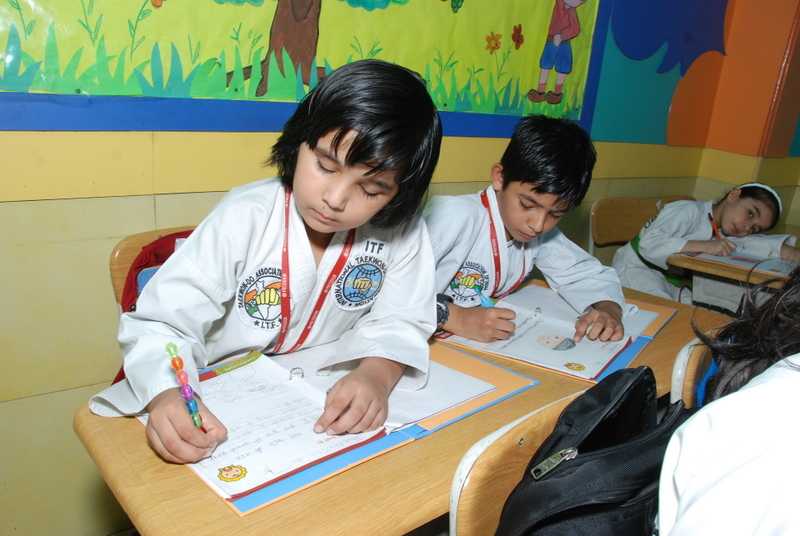TBL

•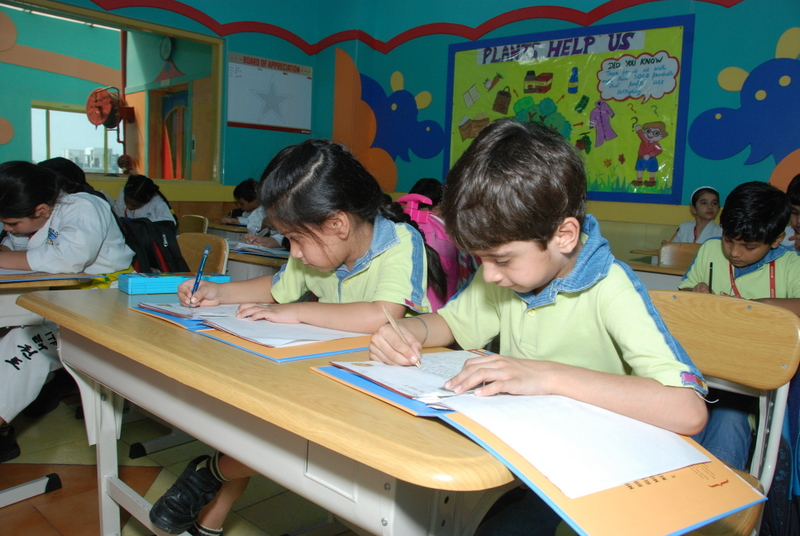TBL

•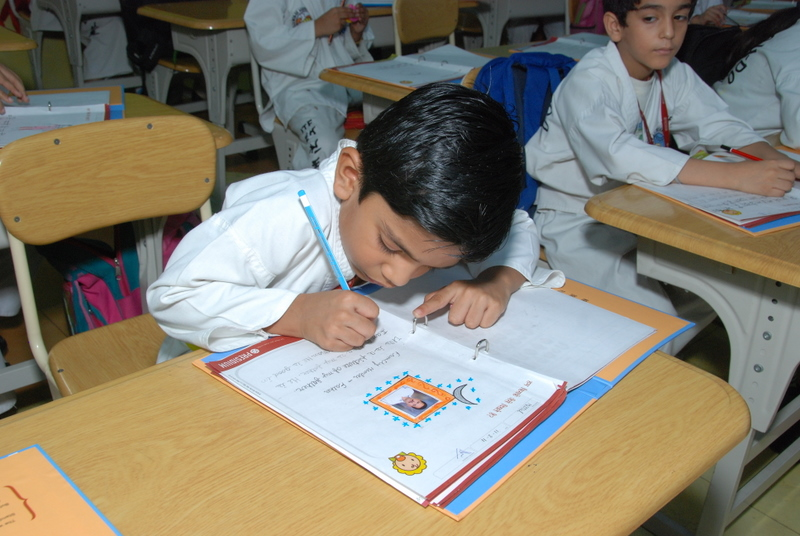TBL

•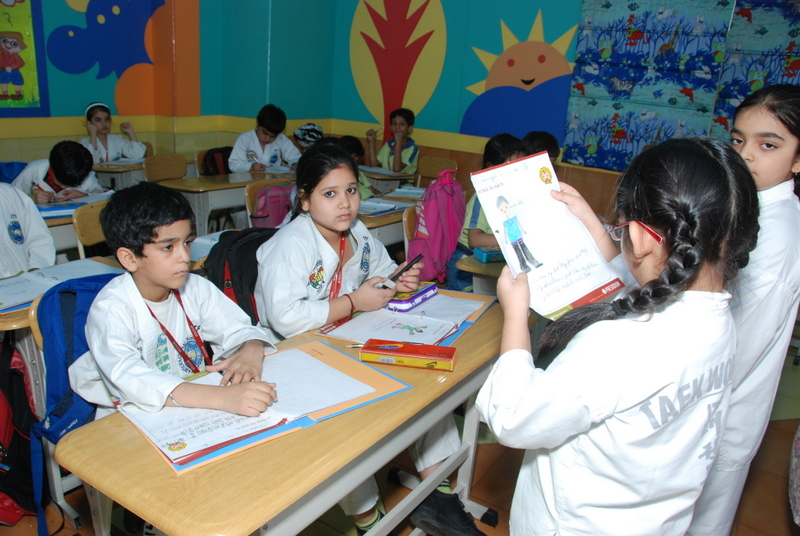TBL

•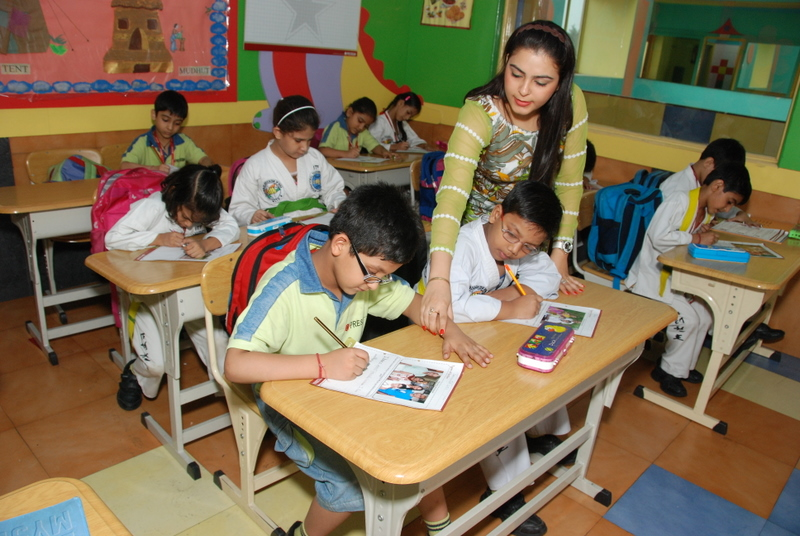TBL

•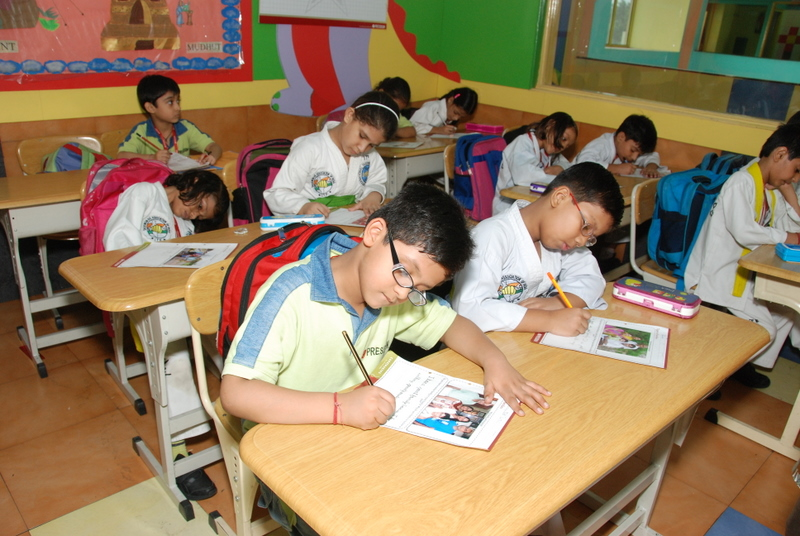TBL

•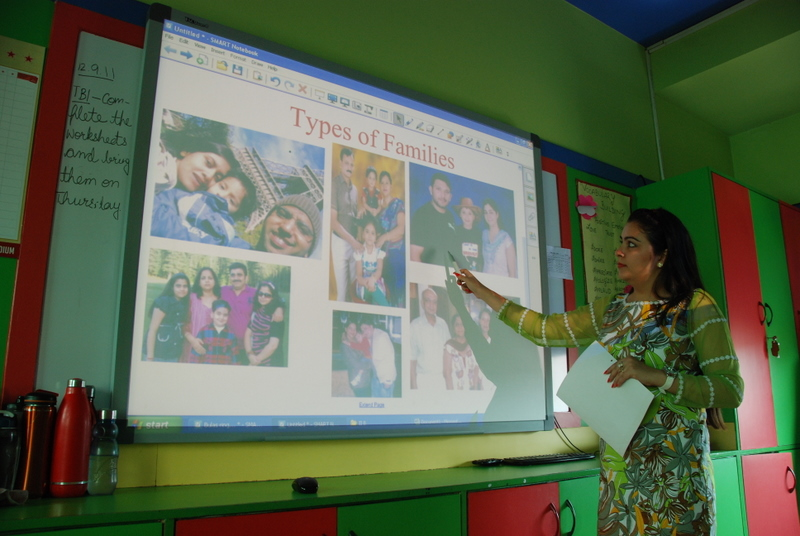TBL

•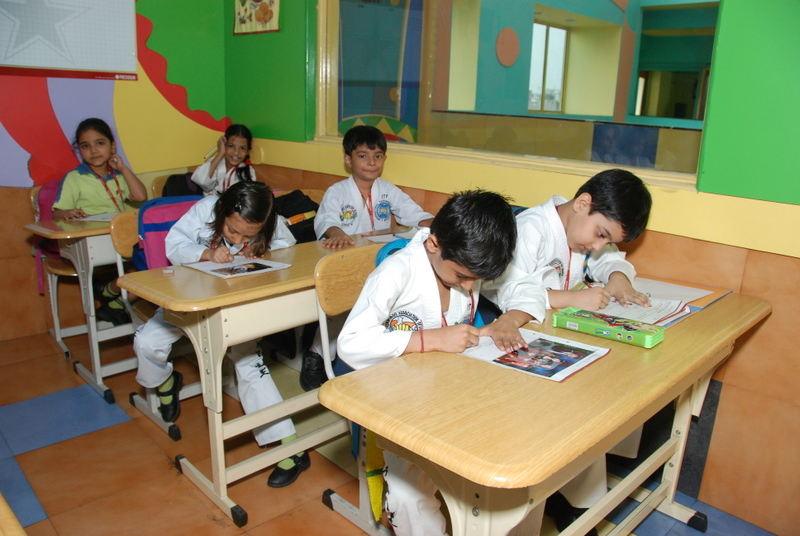TBL

•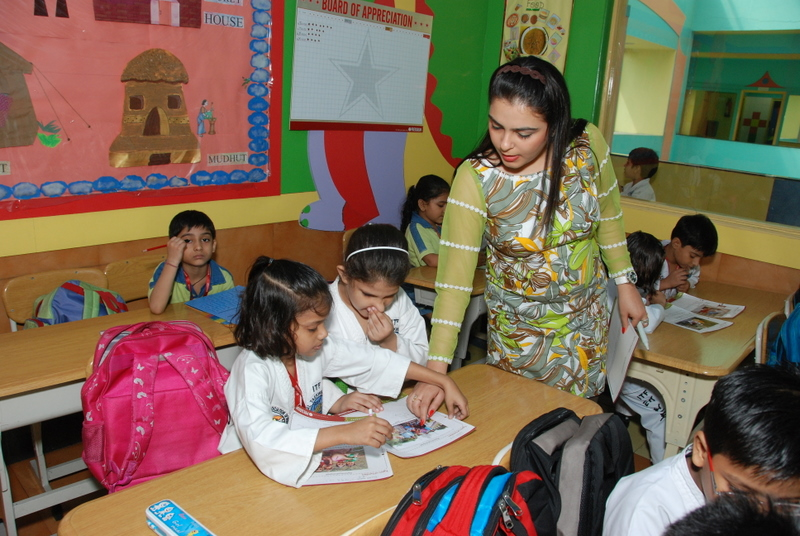TBL

•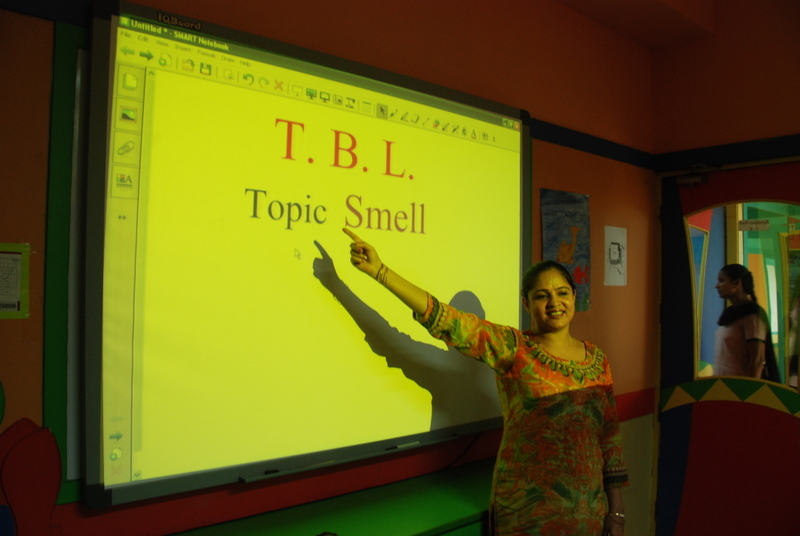TBL

•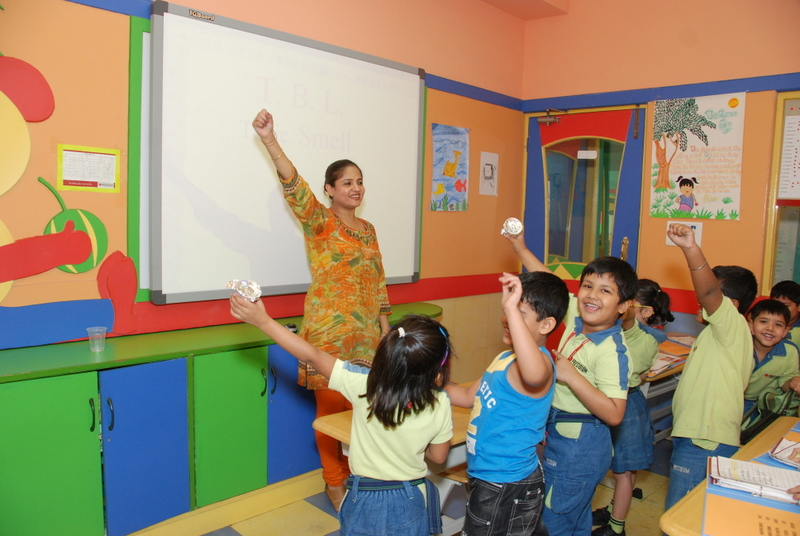TBL

•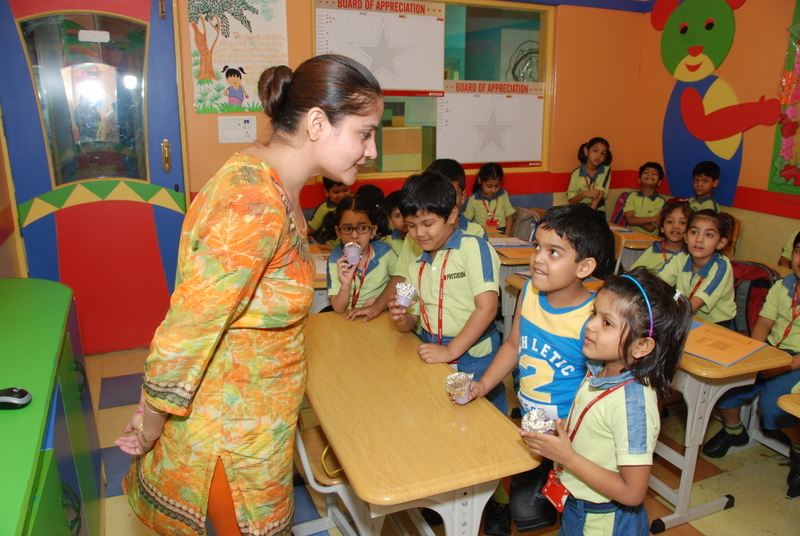TBL

•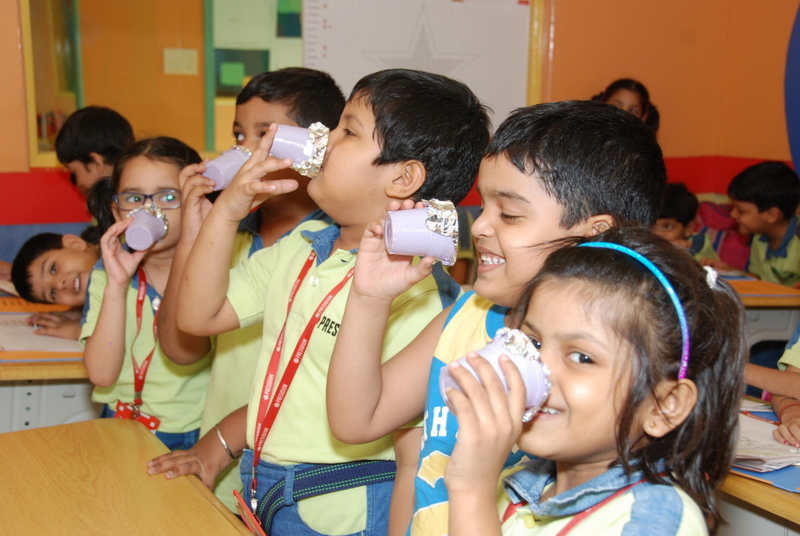TBL

•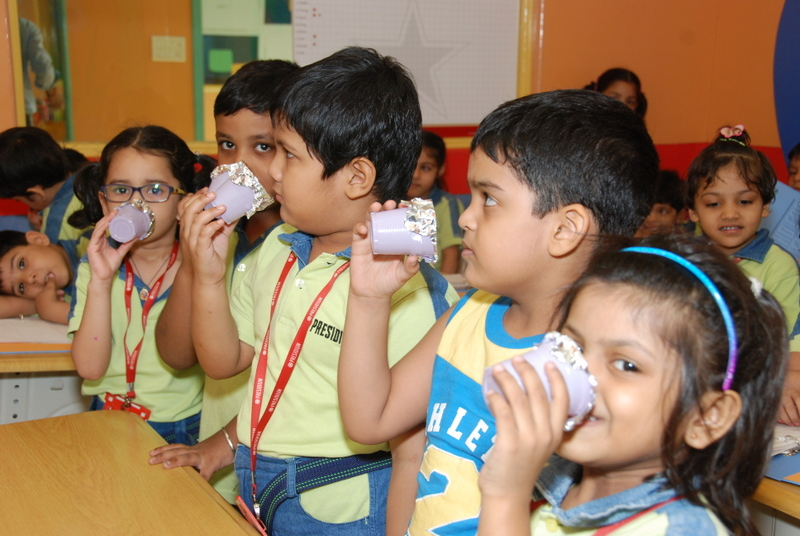TBL

•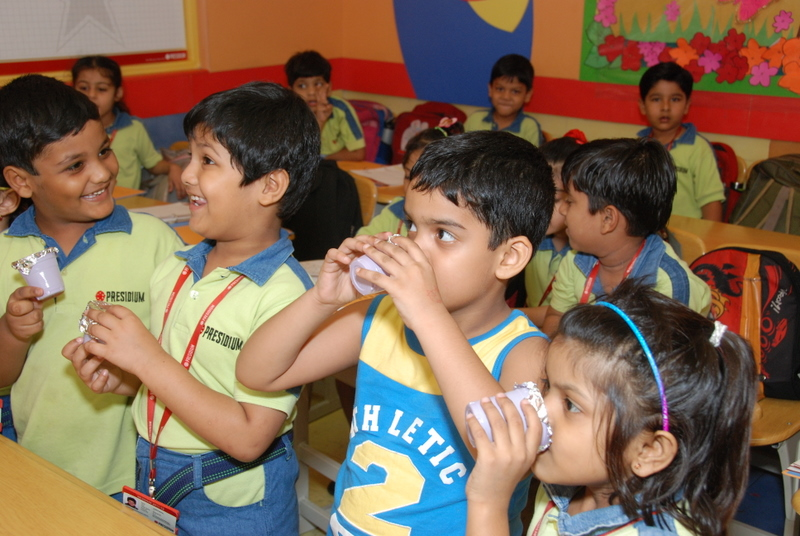TBL

•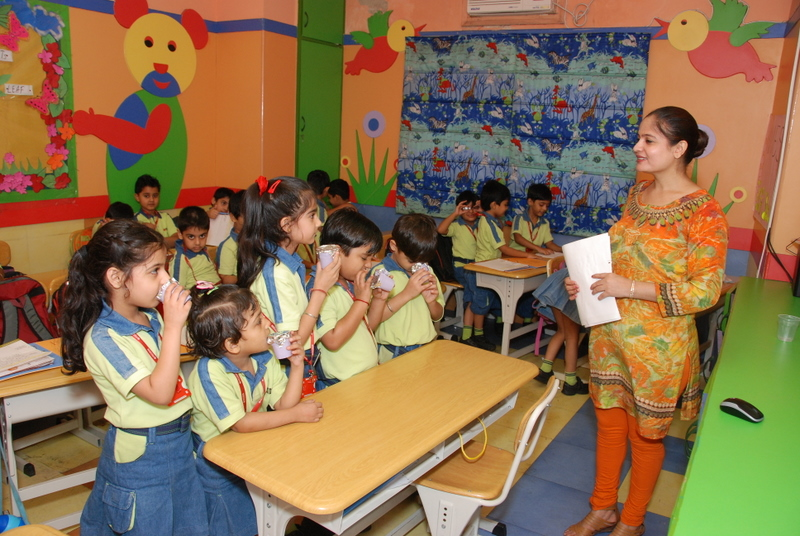TBL

•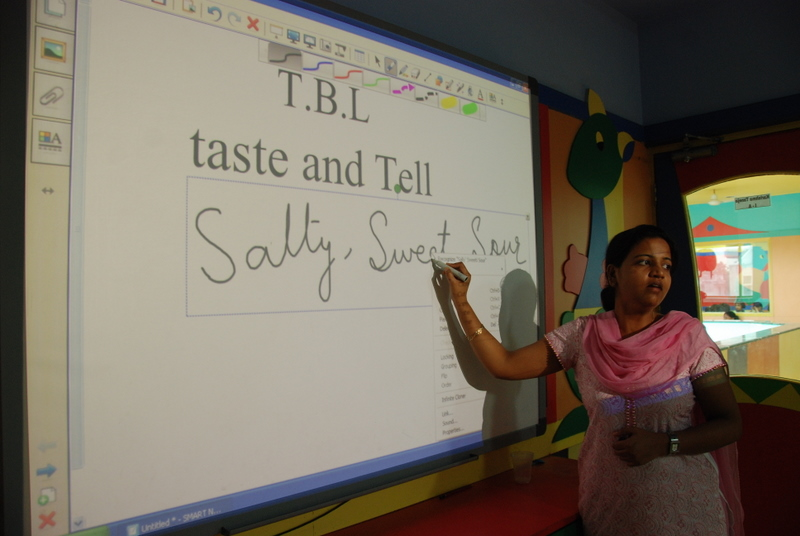TBL

•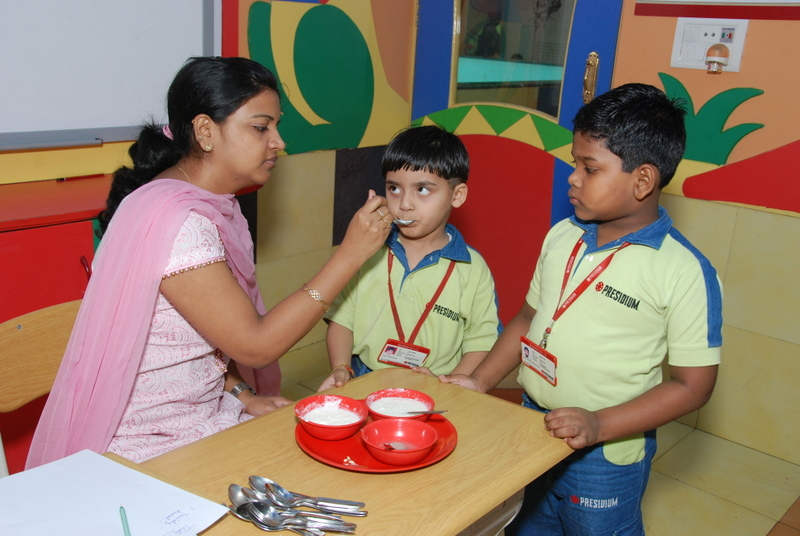TBL

•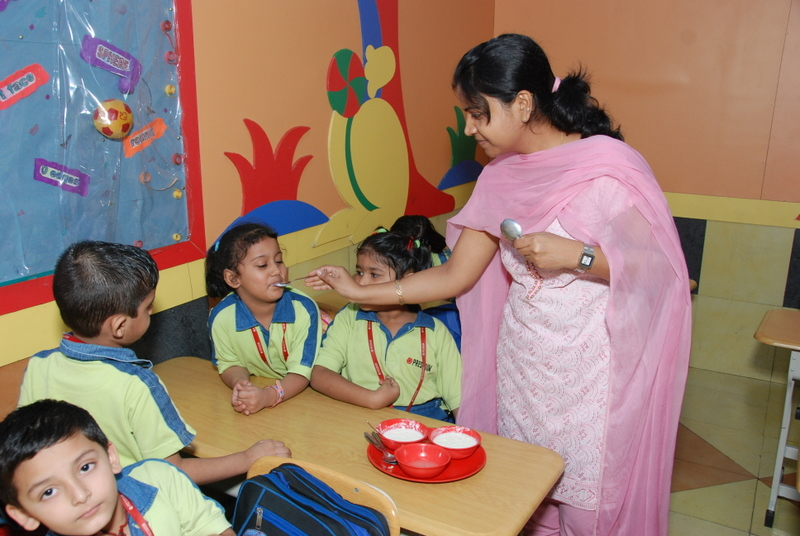TBL

•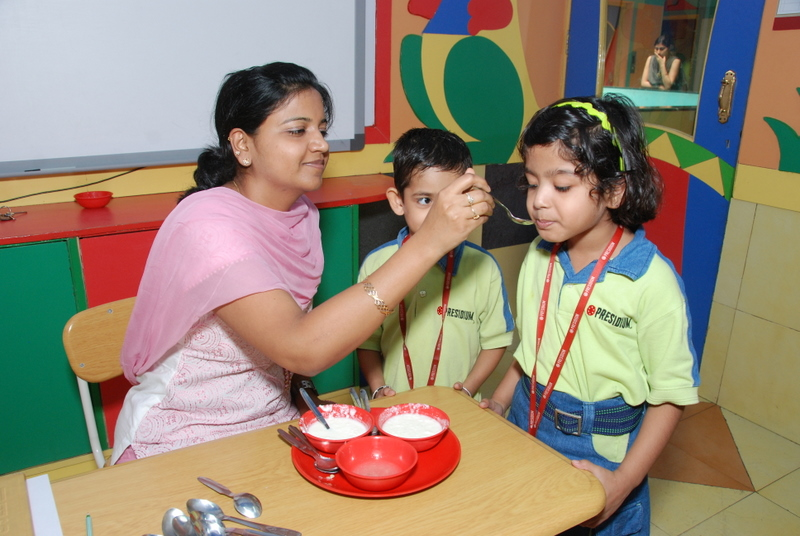TBL